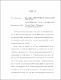## ANALYTIC APPROACHES IN LAGRANGIAN GEOMETRYThe focus of this thesis is two equations that arise in special Lagrangian geometry: the degenerate special Lagrangian equation (DSL) and the Lagrangian mean curvature flow (LMCF). A significant part of this focus centers on Dirichlet duality, subequations, and viscosity solutions, the analytic framework which we use to formulate and study both equations. Given a Calabi--Yau manifold $(X, \omega, J, \Omega)$ and a model manifold $M$, one can construct a kind of moduli space of Lagrangians in $X$ called the space of positive Lagrangians. A Lagrangian $L\subset X$ belongs to this infinite-dimensional space if $L$ is diffeomorphic to $M$ and Re$(\Omega|_L) >0$. % i.e., the set of Lagrangians that are Hamiltonian deformations of a fixed Lagrangian, A Hamiltonian deformation class of the space of positive Lagrangians admits an $L^2$-type Riemannian metric which allows one to study this space from a geometric point of view. Geodesics in this space play a crucial role in a program initiated by Solomon \cite{S1, S2} to understand the existence and uniqueness of special Lagrangian submanifolds in Calabi–Yau manifolds. They also play a key role in a new approach to the Arnold conjecture put forth by Rubinstein–Solomon and in the development of a pluripotential theory for Lagrangian graphs \cite{RS, DR}. The DSL arises as the geodesic equation in the space of positive graph Lagrangians when $X= \mathbb{C}^n$ and $\omega$ and $\Omega$ are associated to the Euclidean structure \cite{RS}. Building on the results of Rubinstein--Solomon \cite{RS}, we show that the DSL induces a global equation on every Riemannian manifold, and that for certain associated geometries this equation governs, as it does in the Euclidean setting, geodesics in the space of positive Lagrangians. For example, geodesics in the space of positive Lagrangian sections of a smooth semi-flat Calabi--Yau torus fibration are governed by the Riemannian DSL on the product of the base manifold and an interval. The geodesic endpoint problem in this setting thus corresponds to solving the Dirichlet problem for the DSL. However, the DSL is a degenerate-elliptic, fully non-linear, second-order equation, and so the standard elliptic theory does not furnish solutions. Moreover, for Lagrangians with boundary the natural domains on which one would like to solve the Dirichlet problem are cylindrical and thus not smooth. These issues are resolved by Rubinstein--Solomon in the Euclidean setting by adapting the Dirichlet duality framework of Harvey--Lawson to domains with corners \cite{RS}. We further develop these analytic techniques, specifically modifications of the Dirichlet duality theory in the Riemannian setting to obtain continuous solutions to the Dirichlet problem for the Riemannian DSL and hence, in certain settings, continuous geodesics in the space of positive Lagrangians. The uniqueness of solutions to the Dirichlet problem in the Euclidean formulation of Dirichlet duality theory relies on an important convex-analytic theorem of Slodkowski \cite{Slod}. Motivated by the significance of this result and the technical, geometric nature of its proof, we provide a detailed exposition of the proof. We then study some of the quantities involved using the Legendre transform, offering a dual perspective on this theorem. Given a Lagrangian submanifold in a Calabi--Yau, a fundamental and still open question is whether or not there is a special Lagrangian representative in its homology or Hamiltonian isotopy class. A natural approach to this problem is the Lagrangian mean curvature flow, which preserves not only the Lagrangian condition but also the homology and isotopy class. Assuming the flow exists for all time and converges, it will converge to a minimal (i.e., zero mean curvature) Lagrangian. In the Calabi--Yau setting these are precisely the special Lagrangian submanifolds. A major conjecture in this area is the Thomas--Yau conjecture \cite{TY}, which posits certain stability conditions on the initial Lagrangian under which the LMCF will exist for all time and converge to the unique special Lagrangian in that isotopy class. Thomas--Yau stated a variant of their conjecture for a related, more tractable flow, called the almost Lagrangian mean curvature flow (ALMCF). In the setting of highly symmetric Lagrangian spheres in Milnor fibers, and under some additional technical assumptions, they make significant progress towards a proof of this variant of the conjecture \cite{TY}. We study the flow of $2$-spheres from a slightly different perspective and provide a relatively short proof of the longtime existence of viscosity solutions under certain stability conditions, and their convergence to a special Lagrangian sphere.